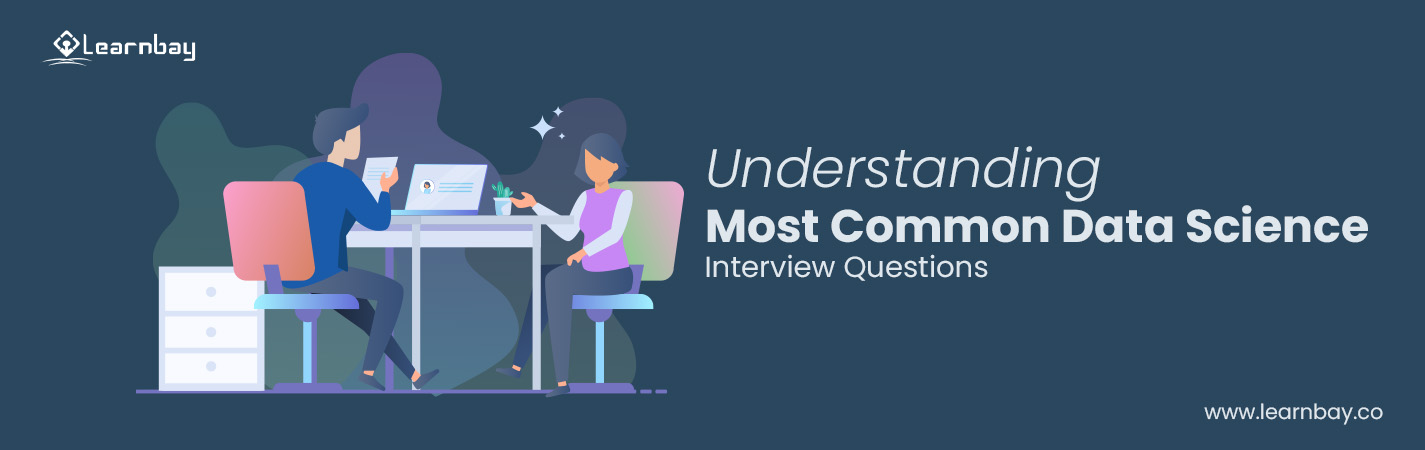# 21 Most Asked Data Science Interview Questions for 2023

By Milan Jain Published in Data Science 15-18 mins

Know the Right Approach to Handle the Most Asked Data Science Interview Questions

Data science is a subject that works on raw data, and the analysis derives patterns that can be used to extract important insights. Usually, the candidates are never worried about completing the course but are more worried about the interview process and the questions asked during it. Professionals are always up for it, and with theoretical knowledge, you need great soft skills too.

Data Science interviews are the toughest to hack is a hoax. If you have worked out problems in classes and have completed all your real-world projects, then half of your work is done. Projects are the main aspect of any data science job, and it would help a lot in an interview as recruiters are more interested to know about your projects than where you have completed your course. Before you go to an interview, there are some questions that you need to be familiar with.

Here is the list ofm basic data science interview questions that are generally asked during a data science interview.

## Questions were mostly asked during a data science interview with top MNCs.

### Supervised Learning :-

1. It uses known and labeled data for input.
2. Supervised Learning includes a Feedback mechanism.
3. Support vector machines, Decision trees, and logistics regression are some of the common algorithms in supervised learning.

### Unsupervised Learning :-

1. Unlabeled data is the input.
2. Feedback mechanism is not included.
3. The common unsupervised learning algorithms are K-means clustering, apriori, and hierarchical clustering.

### 2. How is Logistics Regression Done ?

Estimate probability by using the underlying logistic function. Logistic regression evaluates the connection of the dependent variable(labeling of what you want to predict) and 1 or more independent variables (sigmoid).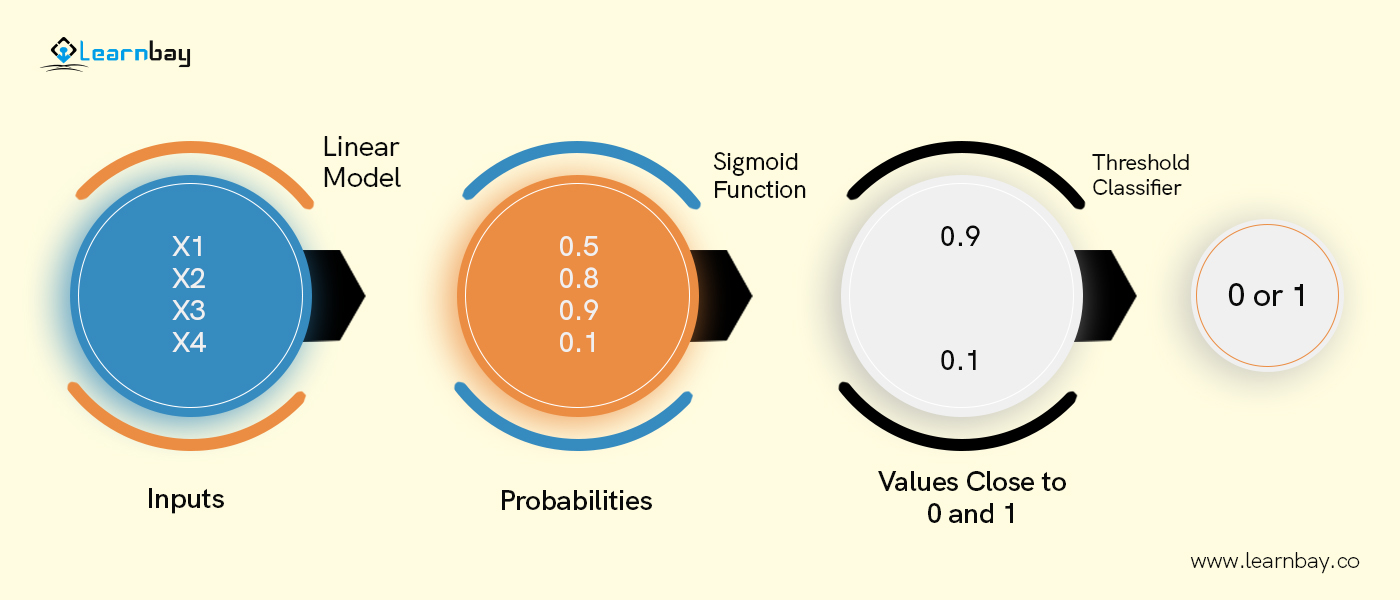The formula and graph for the sigmoid function :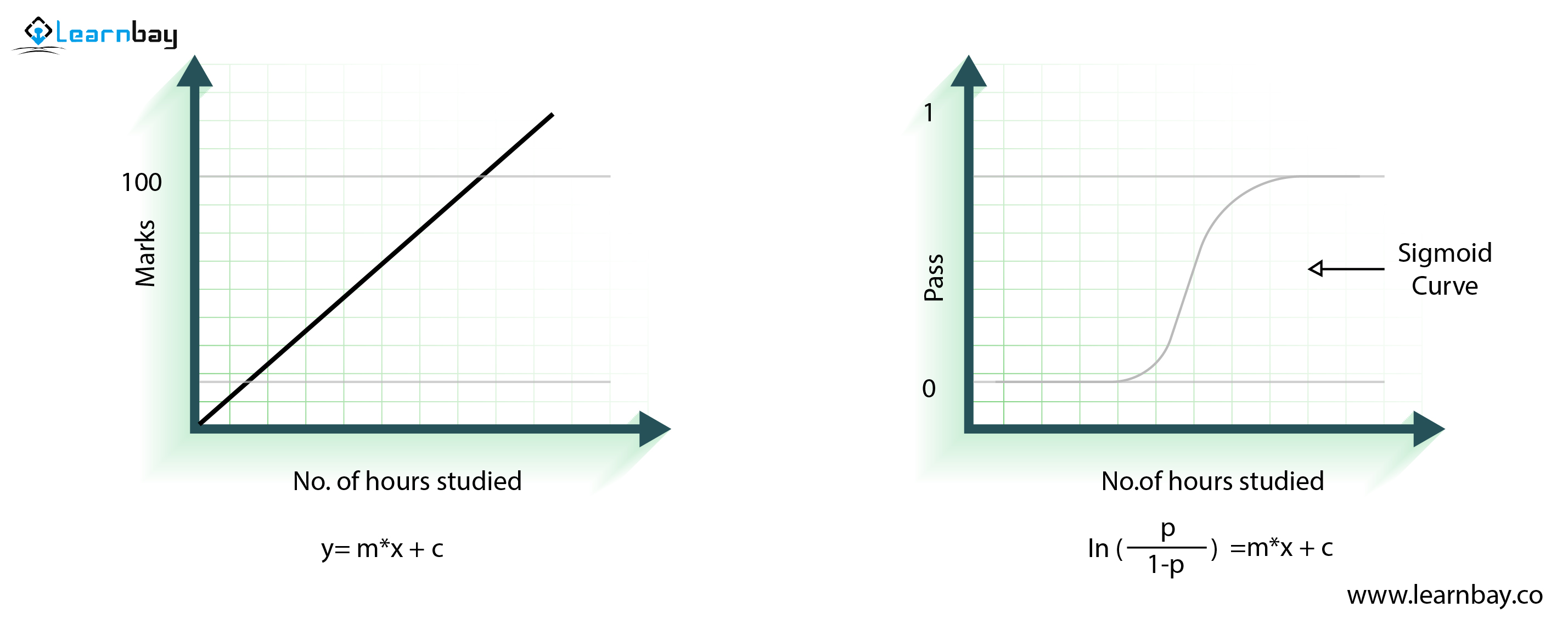### 3. Mention the steps in creating a decision tree

• Consider the whole data as input.
• Calculate target variable entropy and the predictor attributes.
• Calculate the information gained on all attributes.
• You have to take the attribute with the highest information gained as the root node.
• Repeat the above process on every division. So the decision node for every branch is finalized.

### 4. How do you create a model of a random forest ?

A random forest is basically created by multiple decision trees. If you split data differently in packages and create a decision tree in each data group, the random forest will bring all the trees together.

### 5. What are the steps needed to build a random forest model ?

• Select the 'k' feature randomly from the total of 'm' features. (Here k << m)
• In 'K' with the best split point, you can calculate node "D." Split nodes as daughter nodes by best split point.
• Repeat these above steps until there is the finalization of the leaf node.
• By repeating steps 1 & 4 multiple times, create as many trees as you want.

Note : A random sample of n records is drawn from a data set with k records.

### 6. How to avoid overfitting your model ?

An overfitting model is only for a very small amount of data. It ignores big data. There are 3 ways to avoid overfitting :

• Keep a simple model, take a few variables, and remove noise in training data.

• Use K folds for cross-validation.

• Penalize models by regulation techniques like LASSO if they can cause overfitting.

### 7. Differentiate Univariate Bivariate and Multivariate analysis ?

• Univariate

Univariate contains data with one variable and finds patterns from existing data. Learn about the patterns with mean, median, mode, maximum or minimum, dispersion or range, etc.

• Bivariate

Data includes two different variables. Data analysis deals with relationships and causes. This analysis is done to understand the relationship between two variables.

• Multivariate

The data has more than 3 variables. It is divided into multivariate similarly to a bivariate, but it contains more than one dependent variable.

### 8. Which feature selection methods can be utilized to select the right variable ?

Feature selection has two methods :

• Filter :

This involves,

• Linear Discriminant Analysis (LDA)
• Chi-Square test for feature selection
• ANOVA analysis

When you have limited the selected feature, you have to clean the data which is coming in. There is an analogy "Bad data in, Bad data Out."

• Wrapper :

This involves,

• Forward selection : test 1 feature at 1 time and add them to get a good fit.
• Backward selection : Test all the features and then start to remove them to know what works better.
• Recursive Feature elimination : Looks through all different features and how to pair them together.

Wrapper methods are labor-intensive. It requires highly configured computers for more data analysis.

### 9. In your language, write a program that prints numbers ranging from 0-to 50 ?

For 3 multiples, print "Fizz" then the number, 5 multiples, print "buzz ."For 3 and 5 multiples, print "FizzBuzz".

The code is :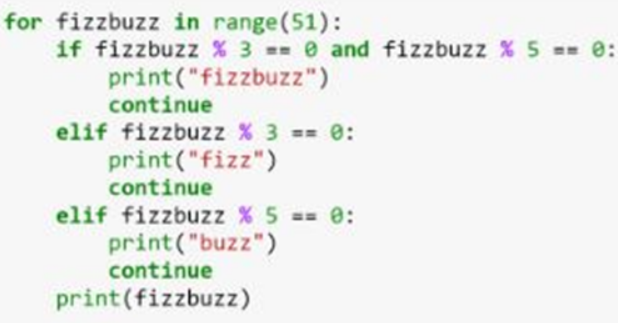### 10. A data set has missing values of 30%. How will you work on it ?

Way to handle missing data values :

When the data is enormous, remove the rows with missing values. It is one of the fastest ways to predict values for the rest of the data.

For smaller data sets, you can opt for substitution on missing values with mean or average for the rest of the data. It is done by working on pandas data frames in Python. You can do it differently, such as df.fillna(mean), and df.mean().

### 11. Calculate the Euclidean distance in Python for the given points ?

plot1 = [1,3]

plot2 = [2,5]

euclidean_distance = sqrt( (plot1-plot2)**2 + (plot1-plot2)**2

### 12. What is dimensionality reduction? Mention its benefits.

It converts vast data set dimensions into fewer dimensions. It is to show similar information simultaneously.

The reduction compresses data and reduces space in storage. It even reduces computation time as few dimensions would lead to less computing. It eliminates redundant features. Like, there is no point in storing a value in two different categories (meter and inches).

### 13. Calculate eigenvalues and eigenvectors 3x3 matrix of the following ?

1 2 2
3 2 1
0 4 2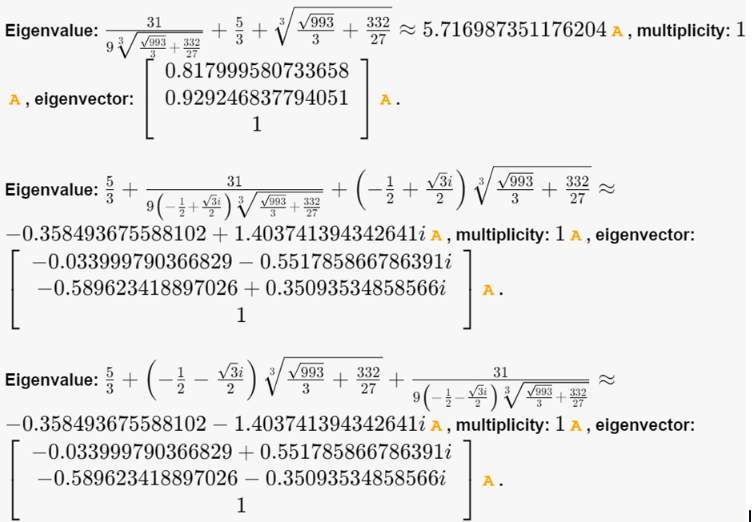### 14. How do you deploy a trained model ?

These are the steps to deploy a trained model :

• Monitor :

Constantly monitoring all models would help to determine performance accuracy. When something changes, you would want to know how the changes will affect other things. It is monitored to see what it is doing and what it has to do.

• Evaluate:

Metrics evaluation of a model is calculated to see if a new algorithm is needed.

• Compare :

The new models would compare to each other. Hence, in the end, it is compared to know which model will give the best performance.

• Rebuild :

Rebuild the current state of data for the best-performing model.

This system gives out the prediction on how a user can rate a specified product based on preferences. It is split into two different areas:

• Collaborative :

In case a recommendation system holds a partial list of listeners' linking orientations, that can generate insight on which type of songs the targeted crowd of listeners would like.

• Content-based :

I.e, Pandora takes the properties of songs to recommend music with the same properties. The content here is more important than who is listening to music. If you listen to 3 classical music out of the 5 songs, then you will receive most of your recommendations in the 'classical' genre.

### 16. Find RMSE and MSE in a linear regression model ?

RMSE and MSE is the measure that is commonly used in a linear regression model. Root mean square error by RMSE.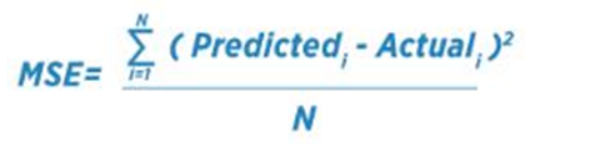The Mean Square Error is highlighted by MSE.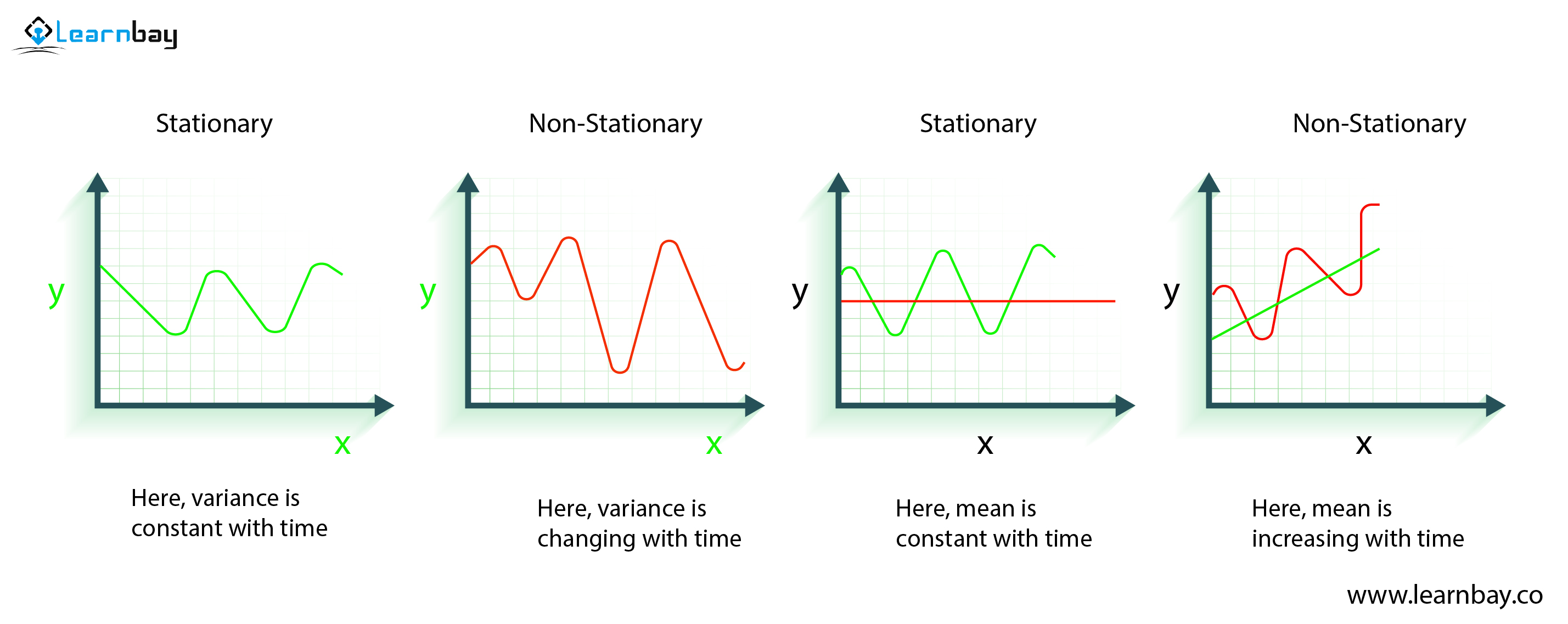### 17. How do you select k for k-means ?

Selecting 'K' for K means clustering can be done by the 'elbow method .'The Elbow method is used to work with K-means clustering of data sets. "K" is the number of clusters. Sum squares (WSS) is the sum of the squared distance between cluster members and the centroid.

# 18. What is the importance of the p-value ?

p-value typically ≤ 0.05

Which shows that there is weak evidence for the null hypothesis. So, you have to reject the null hypothesis.

p-value typically > 0.05

This is indicating strong proof of the null hypothesis. So, approve the null hypothesis.

p-value at cutoff 0.05

It is considered marginal, and it can go either way.

### 19. How to treat Outliers values ?

Drop outliers if they do not have garbage value.

Example : Adults height : ABC ft. It is not true because the height can be a string value. So here, outliers can be eliminated.

If the outliers have extreme value, they can be removed. i.e., if every data point is clustered between 0-10 and one point is 100, then you can remove these points.

In case you cannot drop outliers, try the following:

• Try a different model. Non-linear models detect outliers in data. So, be sure to choose the correct model.

• Normalize data so extreme data points can be pulled to one similar range.

• Algorithms are less affected by outliers.

### 20. How can you declare time-series data as stationery ?

The time-series data is stationary when the series' variance and mean are constant with time.

Here is a visual example :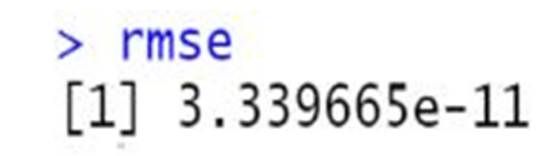The variance is constant with time. X is time, and Y is variable. Y is stationary because it goes through the same points.

The second graph shows the waves are bigger, so it is not stationary, and the variance changes with time.

### 21. How do you calculate accuracy by confusion matrix ?

Consider this

confusion matrix :

The value of all the data, be it actual value or predicted value, can be seen.

The accuracy formula is :

Accuracy = (True Positive + True Negative) / Total Observations

= (262 + 347) / 650

= 609 / 650

= 0.93

We get a result with 93 percent accuracy.

### Summary

These are a few sets of data science basic interview questions along with the shortest possible but impressive answers . This will assist you in your preparation for a data science interview. We will soon post another blog on a different set of data science questions just for you to crack a data science interview.